Next: Signature of a derivative Up: Open problems with signatures Previous: Signature of

## Signature of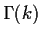The signature ofor at least the signature of k!are very expensive, O(k) operations, to compute. This is not good, as for an unknown x,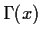would require the computation of Sn(x)! which is O(n). This question in itself is very interesting. Is it possible to compute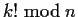in time less than O(k)? This question is open, and very doubtful. If it would be possible, we could do integer factorization in the same time. Non-trivial factors of n can be computed from by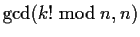. Is it possible to fake the signature of? The only relations between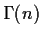and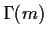are when n-m is an integer and the expression is of size O(|n-m|). Additional care has to be taken with the half argument relations,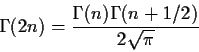its extensions and with the reflection formula.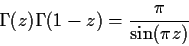Next: Signature of a derivative Up: Open problems with signatures Previous: Signature of
Gaston Gonnet
1999-07-04